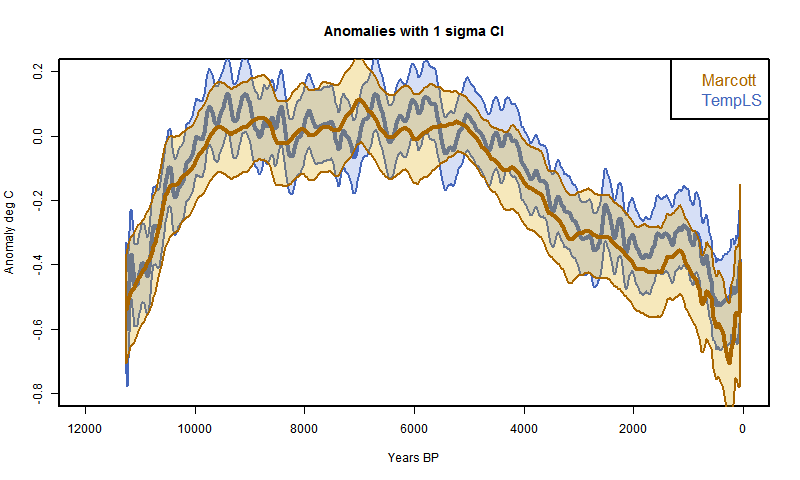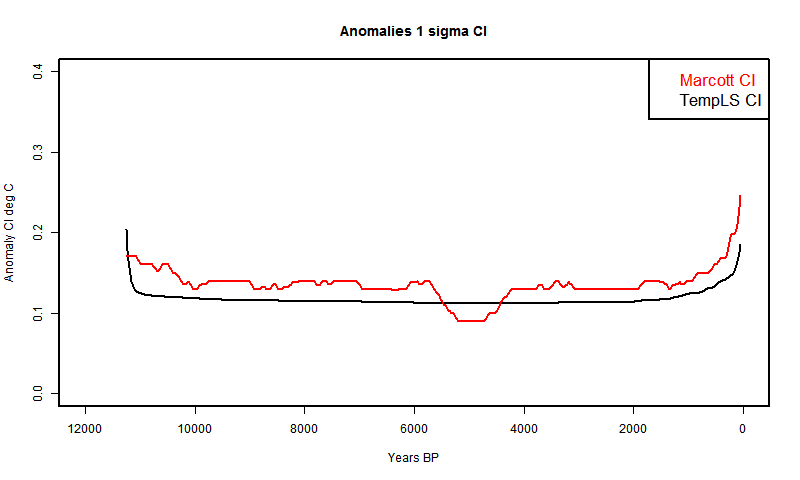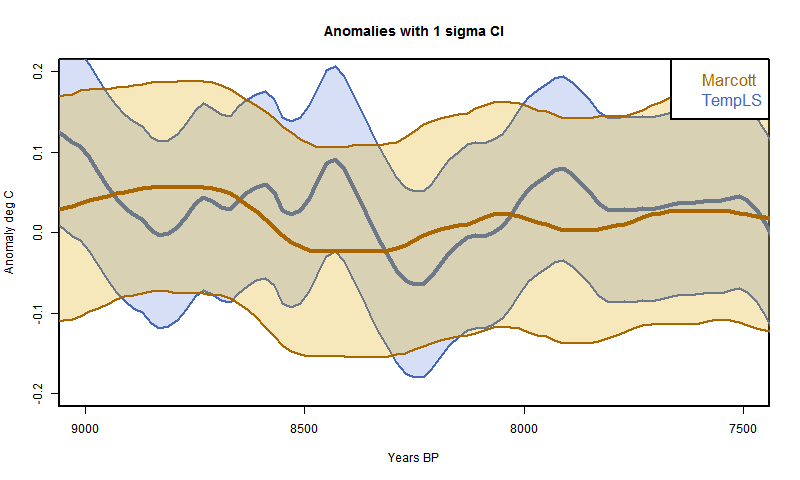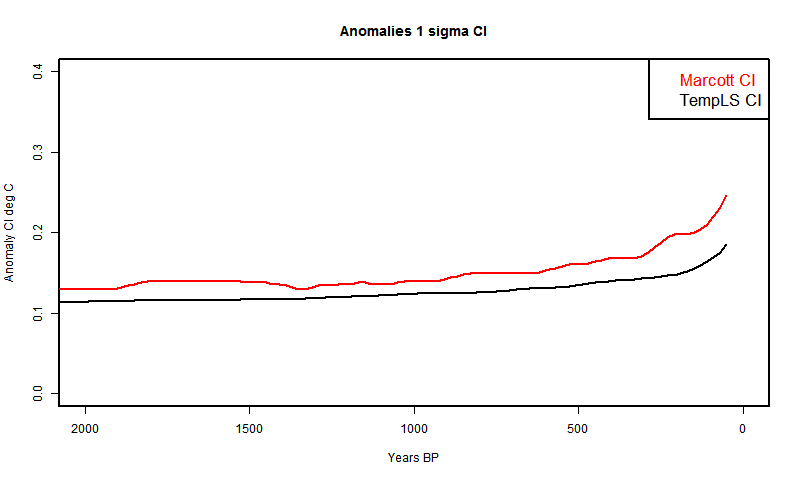## Tuesday, April 16, 2013

### ## TempLS-style paleo recon but with biglm

In the previous post, I described the use of TempLS to fit a reconstruction of temperatures during the Holocene, using the data of Marcott et al 2013. It's actually a rather smaller problem that TempLS normally handles, and can be done by the R package biglm. So I wrote a simpler version for which I can supply code.

To briefly review the TempLS style fitting, the model is that the total set of interpolated proxy temperature readings Ttp can be approximated by a global time function Tt and a set of proxy offsets Pp. The system
Ttp=Tt+Pp
has way more equations than variables, but a least squares solution can be found by regression (biglm).

My interest in doing it this way is that I can use it to implement my next project, which is to make the time expression not a discrete set of points but a projection onto a function space, probably trigs. That will give a more consistent treatment of the limited frequency respoonse, and also have many fewer variables (no interpolation)

The results are very similar to the TempLS results; the CI's are those from biglm. Details below the jump. Plots have been added.

In the lm() formula y~x, y is the listing of all interpolated proxy temperatures; there are about 30000 of them. The x matrix of variables is basically an incidence matrix (no weighting is done in this simple program, but a weight vector could be supplied). There are 565 columns for each time step (20 yrs) and 73 columns for the proxy. Each row has a 1 in the column for time and 1 in the column for proxy. The program consists of compiling this, running biglm, and from the summary using the coefficients and se's to make the plots as in the previous post..

I won't repeat the diagrams, they are very similar to the previous post. The code and data (prox.sav) and plots are in this zipfile.

Update - change of mind. The tracking plots are much the same, but less divergence, and the CI plots are notably smoother. Here they are:And here is the plot with just the CI's for comparison.Here are versions restricted to the last two millenia.